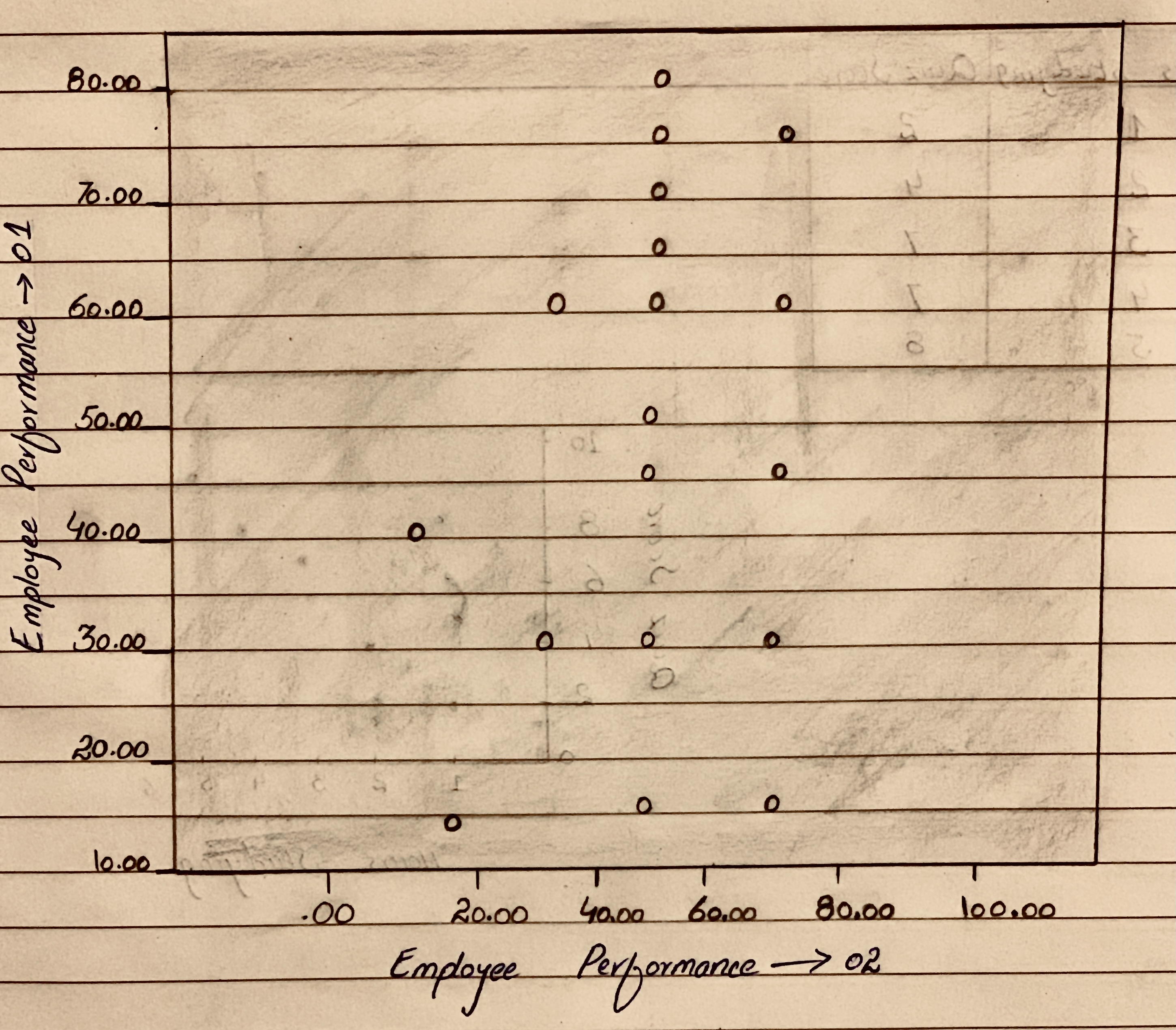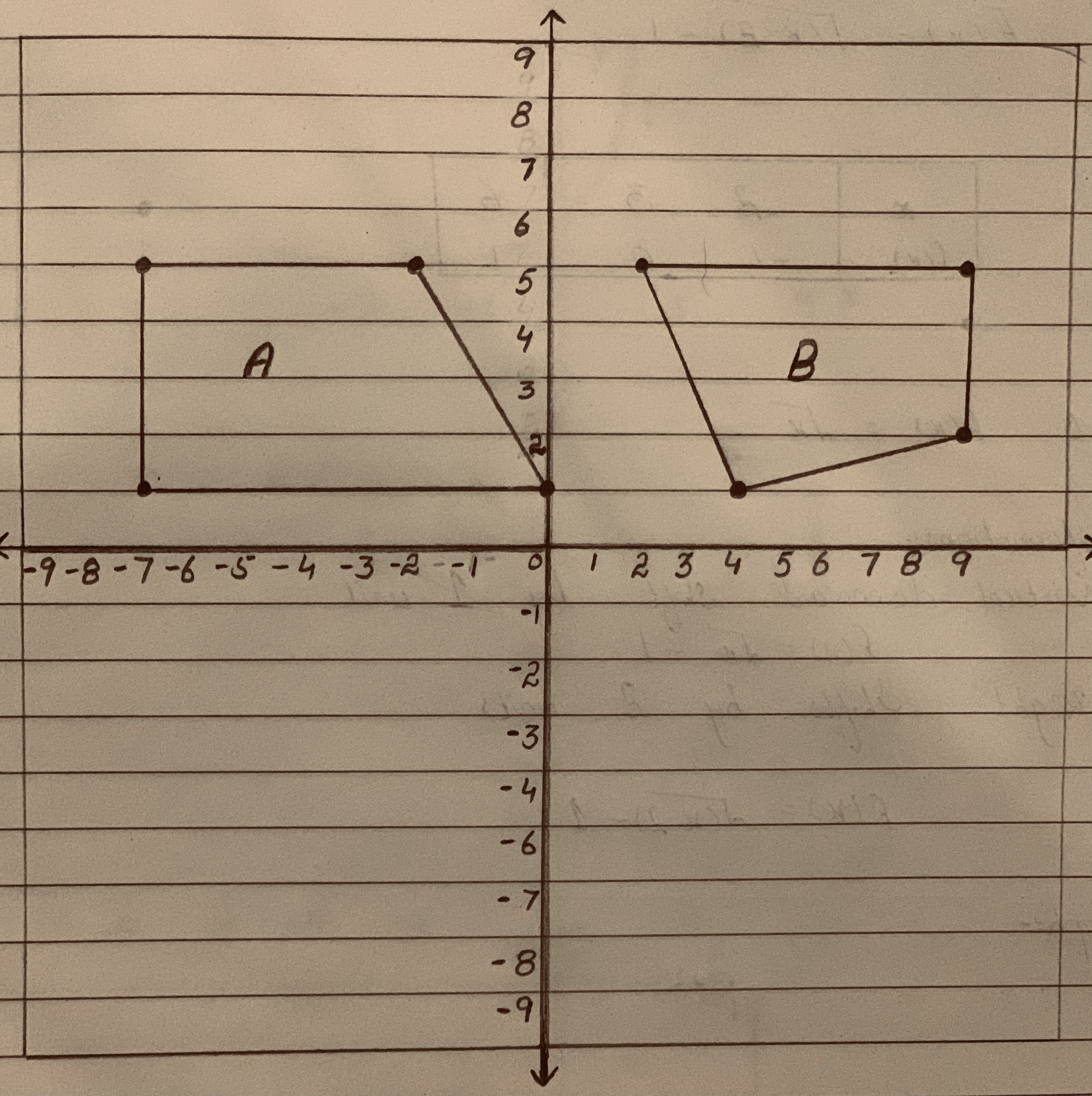# Scatterplots Which of the scatterplots below show a) little or no association? b) a negative association? c) a linear association? d) a moderately strong association? e) a very strong association?Question
ScatterplotsScatterplots Which of the scatterplots below show
a) little or no association?
b) a negative association?
c) a linear association?
d) a moderately strong association?
e) a very strong association?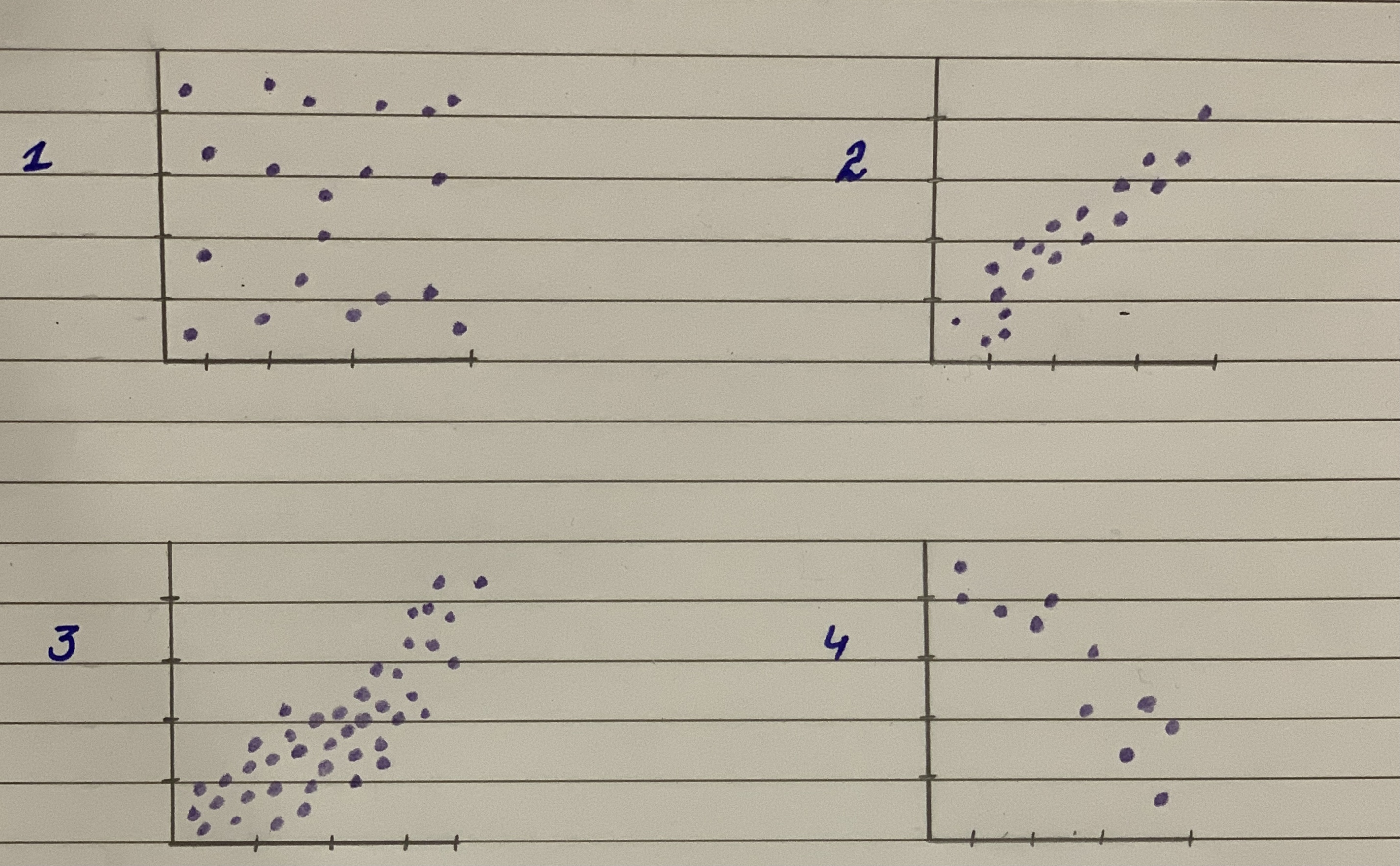2021-01-05
Solution:
a) Little or no association: Scatterplot (1) does not show any pattern. Therefore, it shows little or no association.
b) Negative association: Scatterplot (4) shows a downward tendency pattern. Therefore, it shows a negative association.
c) Linear association: Scatterplot (2) and (4) show upward and downward tendency patterns respectively. Therefore, it shows a linear association.
d) Moderately strong association: Scatterplot (3) shows a parabolic nature. Therefore, it shows a moderately strong association.
e) Strong association: Scatterplot (2) and (4) show a linear upward and downward tendency. Therefore, it shows a very strong association.

### Relevant QuestionsStraight Lines Graph the straight lines in Exercises 1–3.
Then find the change in y for a one-unit change in x, find the point at which the line crosses the y-axis, and calculate the value of y when x 52.5.
1. y x 5 1 2.0 0.5
2. y x 5 1 40 36.2
3. y x 5 25 6
Scatterplots For the scatterplots in Exercises 5 describe the pattern that you see. How strong is the pattern? Do you see any outliers or clusters?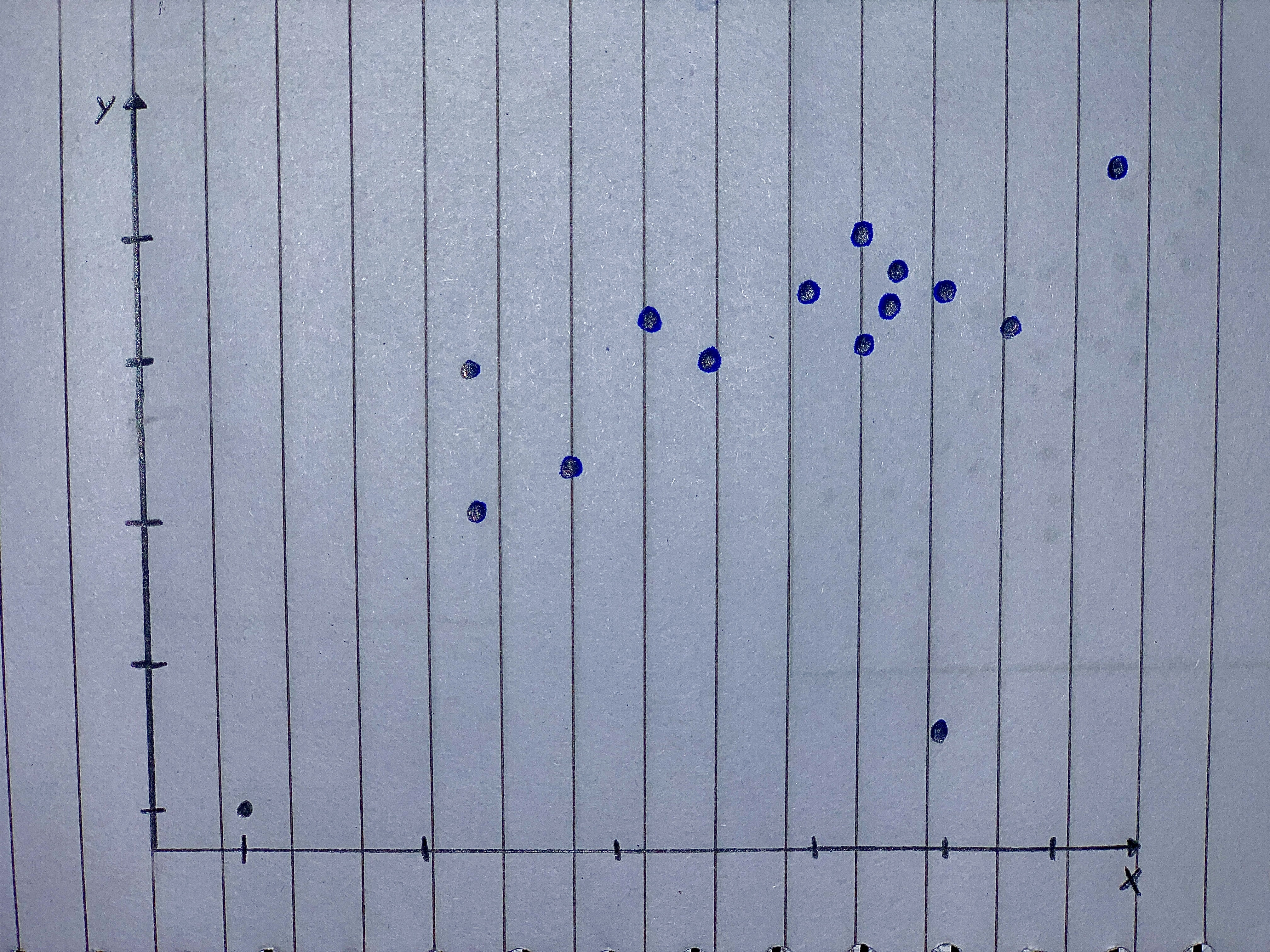Predicting Land Value Both figures concern the assessed value of land (with homes on the land), and both use the same data set. (a). Which do you think has a stronger relationship with value of the land-the number of acres of land or the number of rooms n the homes? Why? b. Il you were trying to predict the value of a parcel of land in e arca (on which there is a home), would you be able to te a better prediction by knowing the acreage or the num- ber of rooms in the house? Explain. (Source: Minitab File, Student 12. "Assess.")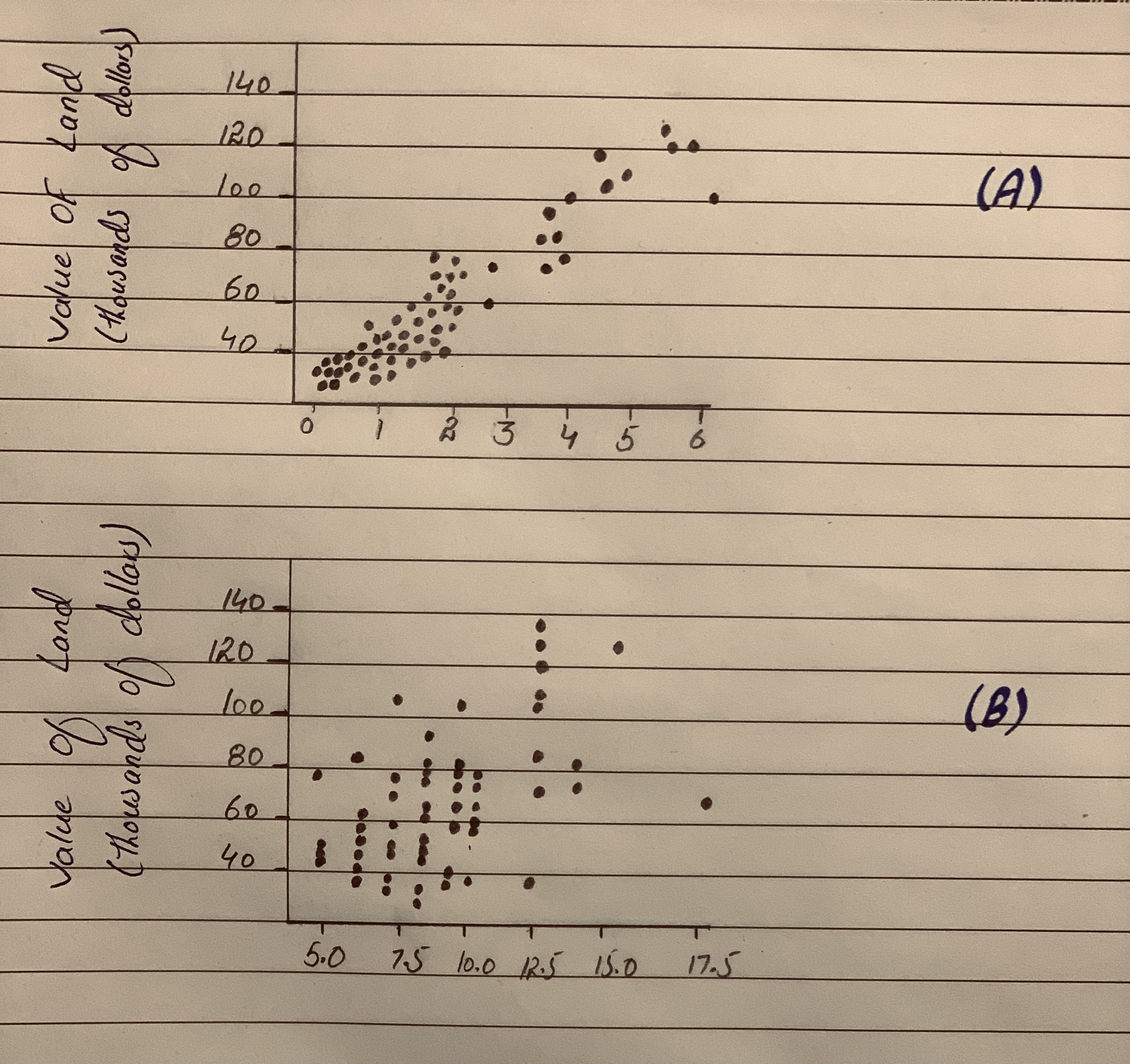Unusual points Each of the four scatterplots that follow shows a cluster of points and one “stray” point. For each, answer these questions:
1) In what way is the point unusual? Does it have high leverage, a large residual, or both?
2) Do you think that point is an influential point?
3) If that point were removed, would the correlation be- come stronger or weaker? Explain.
4) If that point were removed, would the slope of the re- gression line increase or decrease? Explain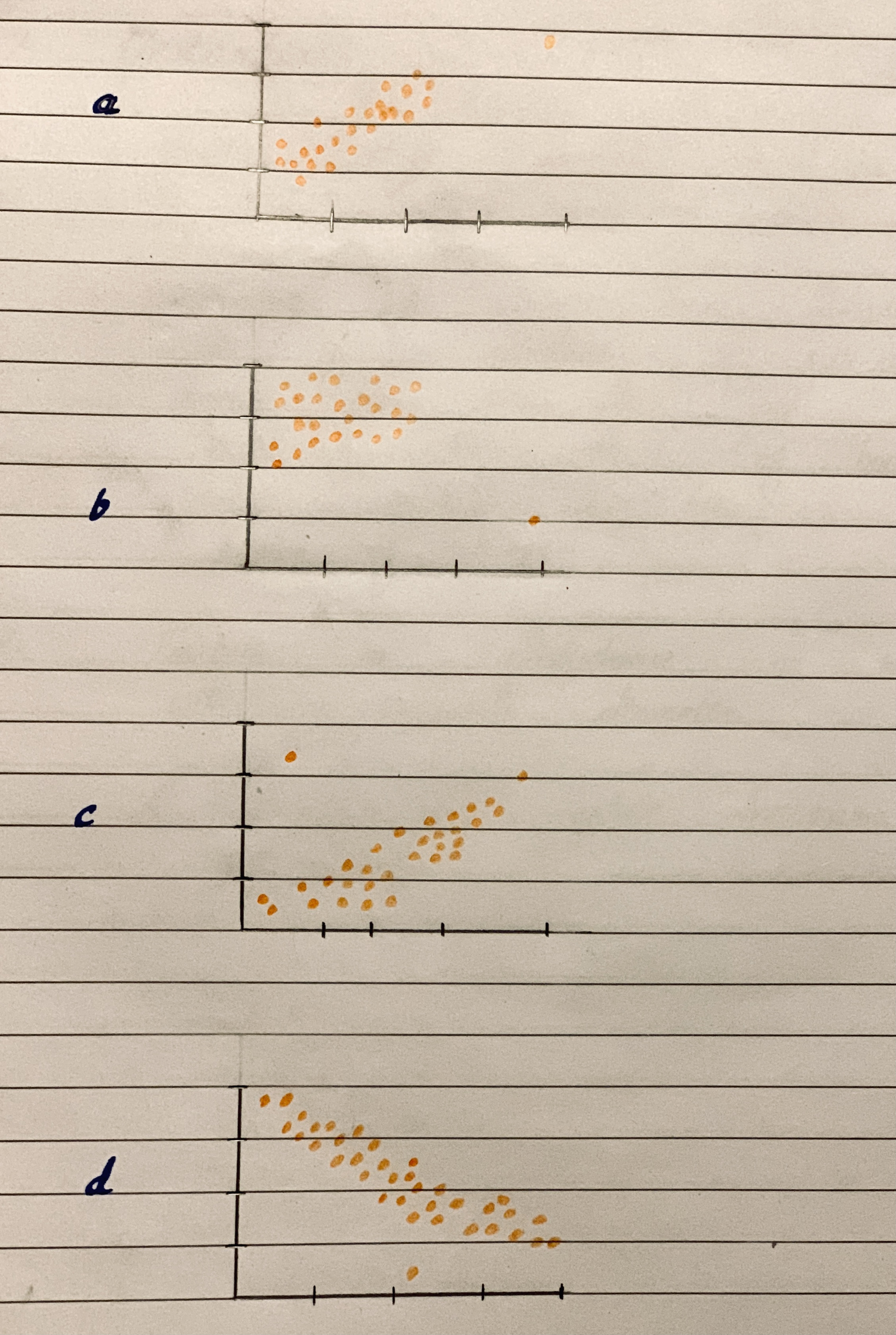Matching Here are several scatterplots. The calculated correlations are -0.923, -0.487, 0.006, and 0.777.
Which is which?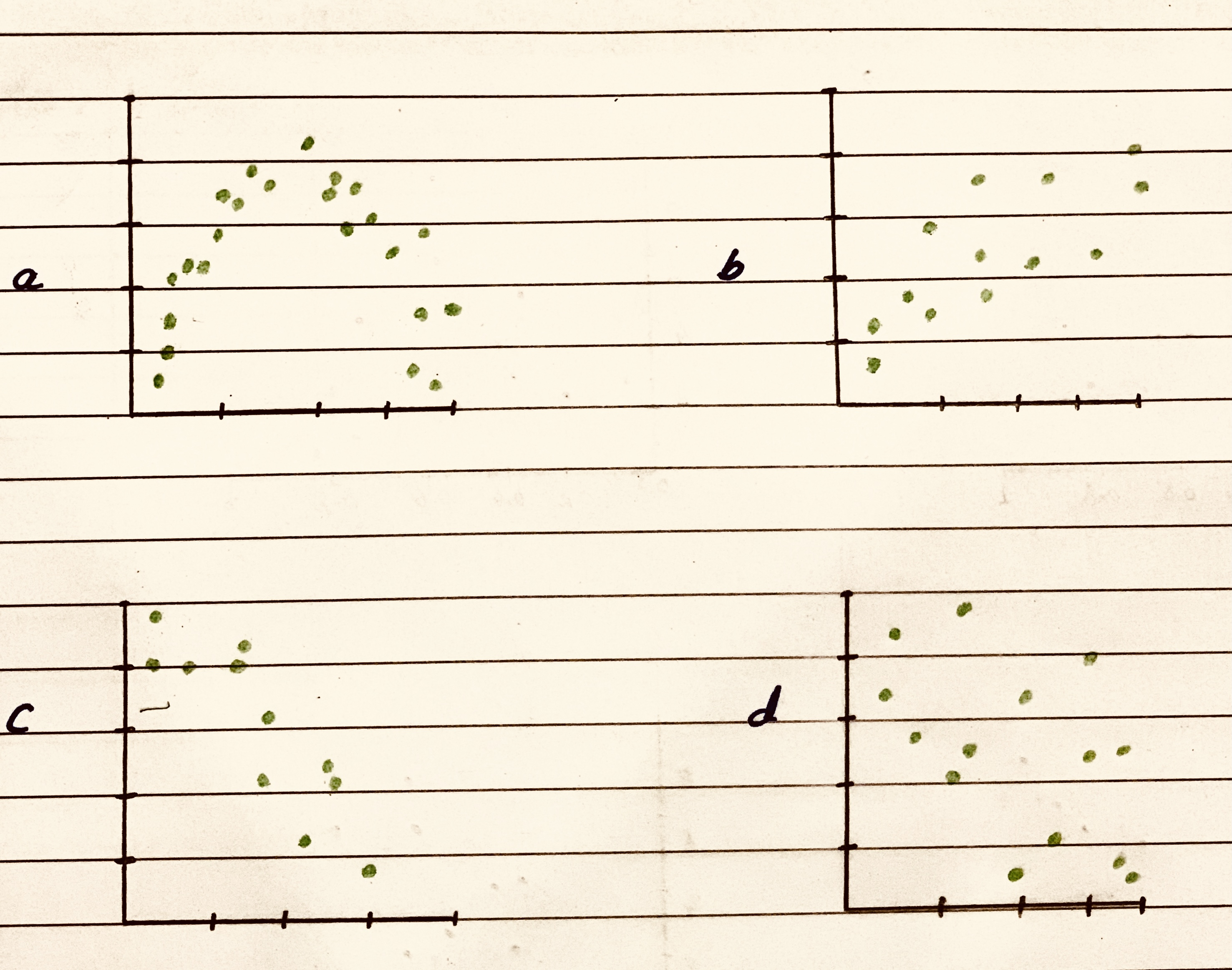Horace keeps track of the amount of time he studies and the score he gets on his quizzes. The data are shown in the table below. Which of the scatter plots below accurately records the data?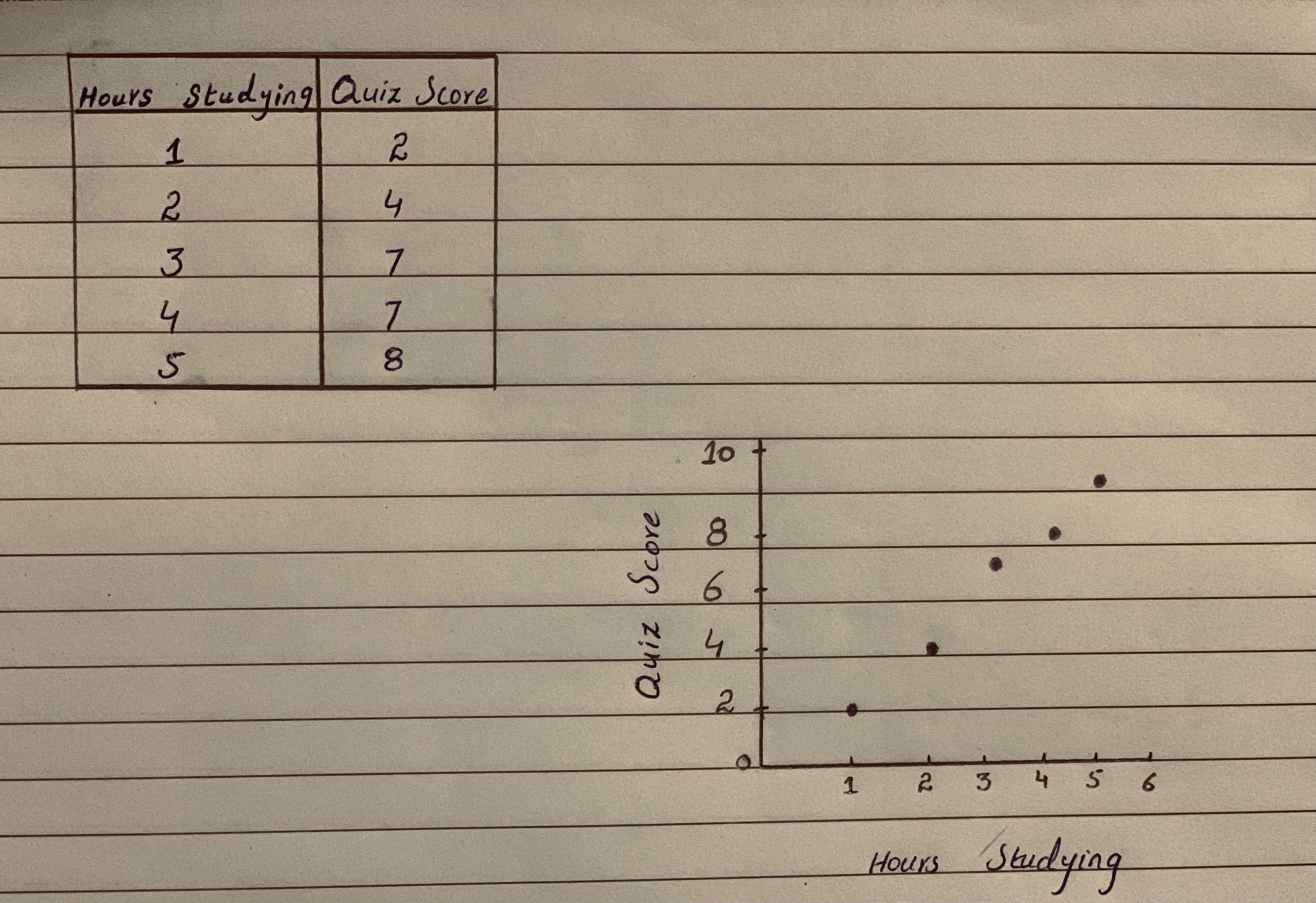Match the values of r with the accompanying scatterplots 0.723,-0.353,0.353,0.998 and -0.998
scatterplots 1,2,3,4,5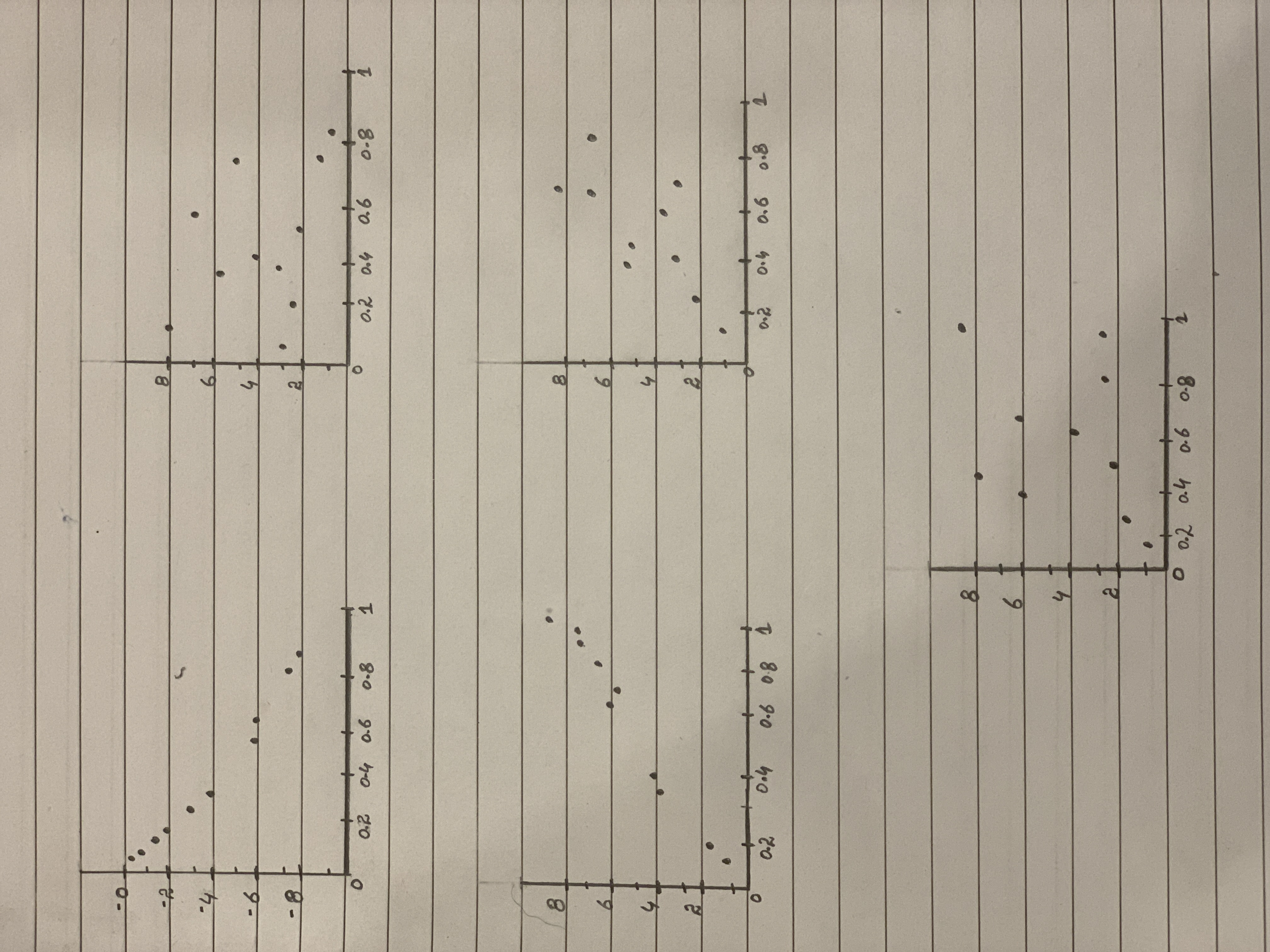Which two of the four scatter plots have most positive correlation and closer to zero correlation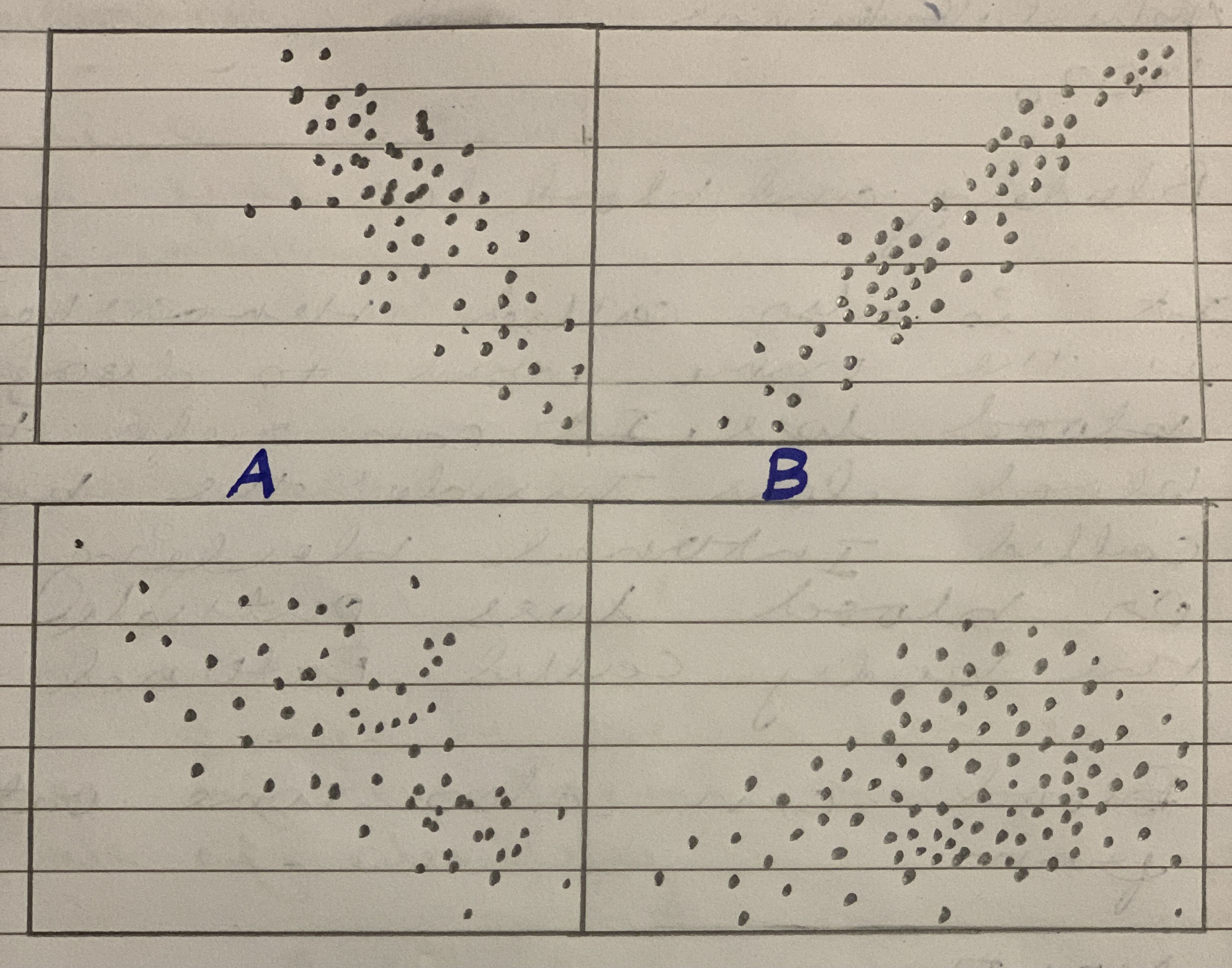Make a scatterplot for each set of data. Tell whether the data show a linear association or a nonlinear association.
(1,2),(7,9.5),(4,7),(2,4.2),(6,8.25),(3,5.8),(5,8),(8,10),(0,0)